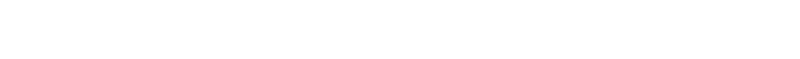### 介绍

```# 导入库
import requests
import nltk
import math
import re
import spacy
import regex as re
import pandas as pd
import numpy as np
import statistics as stats
import matplotlib.pyplot as plt
import matplotlib.cm as cm
import json
# 你需要从NLTK下载一些包。
from bs4 import BeautifulSoup
from nltk import *
from nltk.corpus import stopwords
# #在大多数环境中，你需要安装NER-D。
!pip install ner-d
from nerd import ner
from sklearn.feature_extraction.text import CountVectorizer
from sklearn.feature_extraction.text import TfidfTransformer
from sklearn.cluster import MiniBatchKMeans
from sklearn.feature_extraction.text import TfidfVectorizer```

### 初始化变量

```# 初始化最终数据集的数据字段
dates=[]
titles=[]
locations=[]
people=[]
key_countries=[]
content_text=[]
coord_list=[]
mentioned_countries=[]
keywords=[]
topic_categories=[]
# 为后面的主题模型初始化簇变量
cluster_keywords=[]
cluster_number=[]
# 使用SPACY库初始化NLP对象

### 提取href

```# #从ISW浏览页面获取产品链接
urls=['http://www.understandingwar.org/publications?page={}'.format(i) for i in range(179)]
hrefs=[]
def get_hrefs(page,class_name):
page=requests.get(page)
soup=BeautifulSoup(page.text,'html.parser')
container=soup.find_all('div',{'class':class_name})
container_a=container.find_all('a')
for url in urls:
get_hrefs(url,'view-content')```

### Web爬取

#### 获取所有文本

```# 提取发布数据
def get_date(soup):
try:
data=soup.find('span',{'class':'submitted'})
content=data.find('span')
date=content.get('content')
dates.append(date)
except Exception:
dates.append('')
pass
# 提取产品标题
def get_title(soup):
try:
title=soup.find('h1',{'class':'title'}).contents
titles.append(title)
except Exception:
titles.append('')
pass
# 提取产品的文本内容
def get_contents(soup):
try:
'style','script','body',
'div','a','section','tr',
'aside',]
content=''
text=soup.find_all(text=True)
for t in text:
if t.parent.name not in parents_blacklist and len(t) > 10:
content=content+t+' '
content_text.append(content)
except Exception:
content_text.append('')
pass```

### 自然语言处理

```# 在正文中引用所有国家的列表。
# 如果文本中的一个单词与列表中的一个国家匹配，那幺它将被添加到国家列表中。
def get_countries(content_list):
iteration=1
for i in range(len(content_list)):
print('Getting countries',iteration,'/',len(content_list))
temp_list=[]
for word in word_tokenize(content_list[i]):
for country in country_list:
if word.lower().strip() == country.lower().strip():
temp_list.append(country)
counted_countries=dict(Counter(temp_list))
temp_dict=dict.fromkeys(temp_list,0)
temp_list=list(temp_dict)
if len(temp_list)==0:
temp_list.append('Worldwide')
mentioned_countries.append(temp_list)
# 计算每个国家被提及的次数，然后对照平均值检查每次计数。
# 如果一个国家被提及的次数超过了平均次数，它就会作为一个关键字被记录。
keywords=[]
for key in counted_countries.keys():
if counted_countries[key] > np.mean(list(counted_countries.values())):
keywords.append(key)
if len(keywords) != 0:
key_countries.append(keywords)
else:
key_countries.append(temp_list)
iteration+=1```

### 命名实体识别：地点

#### 找到地名

NLP是机器学习的一种形式，计算机算法使用语法和语法规则来学习文本中单词之间的关系。通过这种学习，NER能够理解某些单词在句子或段落中所起的作用。本教程并不打算全面介绍NLP—对于这样的资源，请查看： https://medium.com/@ODSC/an-i…

#### 从外部API获取坐标

```# 使用NLP提取地名，然后查询open-cage API以获得绘图所需的坐标
# 插入你自己的OpenCage API key:
def get_coords(content_list):
iteration=1
for i in range(len(content_list)):
print('Getting coordinates',iteration,'/',len(content_list))
temp_list=[]
text=content_list[i]
# 应用一个NER算法，从python库'ner-d'中查找地名。
doc=nlp(text)
location=[X.text for X in doc.ents if X.label_ == 'GPE']
location_dict=dict.fromkeys(location,0)
location=list(location_dict)

# 查询位置。

for l in location:
try:
request_url='https://api.opencagedata.com/geocode/v1/json?q={}&key={}'.format(l,geo_api_key)
page=requests.get(request_url)
data=page.json()
for n in range(len(data)):
# 这行代码检查查询结果中的国家是否与mentioned_countries之一相匹配。如果不是，那幺查询结果很可能是假正例。
if data['results'][n]['components']['country'] in mentioned_countries[i]:
lat=data['results'][n]['geometry']['lat']
lng=data['results'][n]['geometry']['lng']
coordinates={'Location': l,
'Lat': lat,
'Lon': lng}
temp_list.append(coordinates)
break
else:
continue
except Exception:
continue
coord_list.append(temp_list)
iteration+=1```

### 命名实体识别：人

#### 验证名字

```def get_people(content_list):
iteration=1
# 使用NER在文本中查找人名。
for i in range(len(content_list)):
print('Getting people',iteration,'/',len(content_list))
temp_list=[]
text=content_list[i]
doc=nlp(text)
persons=[X.text for X in doc.ents if X.label_ == 'PERSON']
persons_dict=dict.fromkeys(persons,0)
persons=list(persons_dict)
full_names=[]
for person in persons:
if len(word_tokenize(person)) >= 2:
string_name=re.sub(r"[^a-zA-Z0-9]+", ' ', person).strip()
full_names.append(string_name)
final_names=[]
for person in persons:
for name in full_names:
tokens=word_tokenize(name)
for n in range(len(tokens)):
if person==tokens[n]:
final_names.append(name)
for name in full_names:
final_names.append(name)
name_dict=dict.fromkeys(final_names,0)
final_names=list(name_dict)
valid_names=[]
for name in final_names:
page=requests.get('https://en.wikipedia.org/wiki/'+name)
if page.status_code==200:
valid_names.append(name)
people.append(valid_names)
iteration+=1```

### 关键词提取：TF-IDF

```# 第一个函数通过降低字符大小写和删除特殊字符对文本进行预处理。
def pre_process(text):
text=text.lower()
text=re.sub("</?.*?>"," <> ",text)
text=re.sub("(\\d|\\W)+"," ",text)
return text
# 这个函数将矩阵映射到坐标。TF-IDF函数将频率分数映射到矩阵，然后需要对这些矩阵进行排序，以帮助我们找到关键字。
def sort_coo(coo_matrix):
tuples = zip(coo_matrix.col, coo_matrix.data)
return sorted(tuples, key=lambda x: (x, x), reverse=True)
# 与上面一样，这是一个帮助函数，一旦频率映射到矩阵，它将帮助排序和选择关键字。
# 这个函数专门帮助我们根据TF-IDF统计数据选择最相关的关键字
def extract_topn_from_vector(feature_names, sorted_items, topn=10):
sorted_items = sorted_items[:topn]
score_vals = []
feature_vals = []
for idx, score in sorted_items:
fname = feature_names[idx]
score_vals.append(round(score, 3))
feature_vals.append(feature_names[idx])
results= {}
for idx in range(len(feature_vals)):
results[feature_vals[idx]]=score_vals[idx]
return results
#最后一个函数包含了上述helper函数，它对正文应用TF-IDF算法，根据使用频率查找关键字。
def get_keywords(content_list):
iteration=1
processed_text=[pre_process(text) for text in content_list]
stop_words=set(stopwords.words('english'))
cv=CountVectorizer(max_df=0.85,stop_words=stop_words)
word_count_vector=cv.fit_transform(processed_text)
tfidf_transformer=TfidfTransformer(smooth_idf=True,use_idf=True)
tfidf_transformer.fit(word_count_vector)
feature_names=cv.get_feature_names()
for i in range(len(processed_text)):
print('Getting Keywords',iteration,'/',len(content_list))
doc=processed_text[i]
tf_idf_vector=tfidf_transformer.transform(cv.transform([doc]))
sorted_items=sort_coo(tf_idf_vector.tocoo())
keys=extract_topn_from_vector(feature_names,sorted_items,10)
keywords.append(list(keys.keys()))
iteration+=1```

### 主题模型

NLP中最常见的任务之一就是主题模型。这是一种聚类形式，它尝试根据文档的文本内容自动对文档进行分类。在这个具体的例子中，我想一眼就知道ISW涉及哪些主题。通过根据文本内容对文档进行分类，我可以轻松地对文档的主要思想有一个大致的了解。

#### 聚类

```# 该函数根据各种“k”参数检查聚类算法，以找到“k”的最优值。
def find_optimal_clusters(data, max_k):
iters = range(2, max_k+1, 2)

sse = []
for k in iters:
sse.append(MiniBatchKMeans(n_clusters=k,
init_size=1024,
batch_size=2048,
random_state=20).fit(data).inertia_)

print('Fit {} clusters'.format(k))

f, ax = plt.subplots(1, 1)
ax.plot(iters, sse, marker='o')
ax.set_xlabel('Cluster Centers')
ax.set_xticks(iters)
ax.set_xticklabels(iters)
ax.set_ylabel('SSE')
ax.set_title('SSE by Cluster Center Plot')
# 从内容列表中获取关键词来帮助对主题模型的分类
def get_top_keywords(data, clusters, labels, n_terms):
df = pd.DataFrame(data.todense()).groupby(clusters).mean()
for i,r in df.iterrows():
cluster_keywords.append(','.join([labels[t] for t in np.argsort(r)[-n_terms:]]))

# 应用于主题建模的内容列表
def get_topics(content_list):
processed_text=[pre_process(text) for text in content_list]
stop_words=set(stopwords.words('english'))
cv=CountVectorizer(max_df=0.85,stop_words=stop_words)
word_count_vector=cv.fit_transform(processed_text)
tfidf_transformer=TfidfTransformer(smooth_idf=True,use_idf=True)
tfidf_transformer.fit(word_count_vector)
feature_names=cv.get_feature_names()
vector=tfidf_transformer.transform(cv.transform(processed_text))
#find_optimal_clusters(vector,50)
clusters = MiniBatchKMeans(n_clusters=50, init_size=1024, batch_size=2048, random_state=20).fit_predict(vector)
for cluster in clusters:
cluster_number.append(int(cluster))
get_top_keywords(vector, clusters, cv.get_feature_names(), 20)```

### 放在一起

```# 遍历从“browse”中提取的href，提取相关内容
iteration=1
# 前几个函数依赖于原始提取的web内容作为参数。这些都是基本的web抓取技术。
for href in hrefs:
print('Web scraping: iteration',iteration,'/',len(hrefs))
page=requests.get(href)
soup=BeautifulSoup(page.text,'html.parser')
get_date(soup)
get_title(soup)
get_contents(soup)
iteration+=1
# 下面这些函数依赖于文本主体作为参数。
# 这些是基于nlp的函数。
# 注意:由于查询外部API，
# 需要一个超时来阻止服务器过载。
# 这部分代码的运行时间很长。
get_countries(content_text)
get_coords(content_text)
get_people(content_text)
get_keywords(content_text)
get_topics(content_text)```

### 丰富主题模型```#搜索与主题对应的关键词列表，与聚类词库交叉引用，为每篇文章分配一个主题类别。
'caliphate','isis','terrorist','terrorism']
'idlib','afrin','aleppo']
russia=['Russia','russia','belarus','slavic','kremlin','russian',
'minsk','ukraine','putin']
iran=['Iran','iran','iranian','proxy','militias','militia','marjah']
turkey=['Turkey','erdogan','turkish','turkey']
ors=['ORS','kabul','ghani','pakistan','afghan','afghanistan',
'taliban','ansf','karzai','helmand']
africa=['Africa','libya','libyan','egypt','egyptian','africa','african']
cat_list=[oir,terrorism,syrian_conflict,russia,iran,turkey,ors,africa]
topic_dict={}
for i in range(len(cluster_keywords)):
temp_list=[]
for n in nltk.word_tokenize(cluster_keywords[i]):
for item in cat_list:
if n in item:
temp_list.append(item)
temp_dict=dict.fromkeys(temp_list,0)
temp_list=list(temp_dict)
topic_dict[i] = temp_list
for num in cluster_number:
topic_categories.append(topic_dict[num])```

### 数据库创建

```#将一个空列表初始化
db=[]
for i in range(len(hrefs)):
countries={
'focus area': key_countries[i],
'all mentioned countries': mentioned_countries[i]
}
# 将函数中定义的所有列表添加到新的存储列表中
doc={
'_id': len(hrefs) - i,
'title': titles[i],
'date': dates[i],
'places': coord_list[i],
'people': people[i],
'keywords': keywords[i],
'countries': countries,
'full text': content_text[i],
'topic cluster': cluster_number[i],
'categories': topic_categories[i]
}
db.append(doc)
# 将列表保存为谷歌驱动器内的.JSON数据存储文件(用于演示目的)
with open ('/content/drive/My Drive/Colab Notebooks/isw_products.json', 'w') as fout:
json.dump(db, fout)```

### 摘要